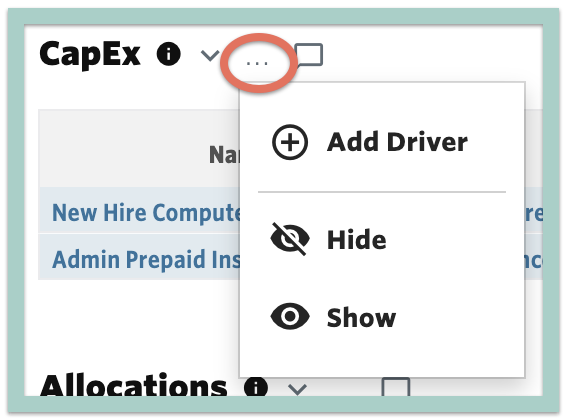Help Center
1. Help Center
2. Plans
3. Balance Sheet and Cash Planning

# CapEx Assets & Drivers

## Documentation for planning capital expenditures using the CapEx module

Capital Expense planning is done through the CapEx module. This module has two associated tables, CapEx Asset & CapEx, both of which are located in Plans > Drivers.

The CapEx Asset table allows you to define the characteristics of the asset to be purchased and the CapEx table determines when that asset should be purchased.

The output of this module will incrementally increase the Fixed Asset Balance Sheet account. It will also calculate the depreciation schedule and map it to both the periodic expense line item on the Income Statement as well as the accumulated deprecation on the Balance Sheet.

In addition to Capital Expenditures, this module can be used to plan any amortizable asset, e.g., Prepaid expense.

### CapEx Assets

Located in Plans > Drivers, this table is used to define the characteristics of the asset to be purchased.

To add a new CapEx Asset, select the ... to the right of the CapEx Assets header and select + New Asset.The fields available to define for the CapEx Asset are as follows:

• Name - specify the name which is referenced in the Asset field for the CapEx Driver
• Asset Account - choose the balance sheet account which should increase when the asset is purchased
• Asset Contra Account - choose the balance sheet account that should reflect the accumulated depreciation for the asset
• Expense Account - choose the income statement account that should reflect the periodic depreciation of the asset
• Expense Department - choose the income statement department that should reflect the periodic depreciation of the asset
• Amort Period - enter in months the asset should be amortized or depreciated over, e.g., if the asset has a useful life of 3 years enter 36
• Asset Cost - input the expected purchase price of the asset
• Residual Value - optionally enter the future value of the asset that you believe you can sell the asset for at the end of the assets life or amortization period
• Amort Delay - optionally input a delay in calculating the amortization after the purchase date, e.g., enter a 3 if you plan to put the asset in service 3 months after the purchase date
• Amortized Value - read-only system calculation that represents the total amount of the asset that will be amortized - it is calculated as Asset Cost - Residual Value
• Monthly Amortization - read-only system calculation that represents the monthly amortization reflected on the income statement & balance sheet - it is calculated as Amortized Value / Amort Period

### CapEx Driver

Located in Plans > Drivers, this table is used to trigger a CapEx Asset to be purchased in the plan.

To add a new CapEx driver, select the ... to the right of the CapEx header and select + New Driver.The fields available to define for the CapEx driver are as follows:

• Name - free text field - use a name that is meaningful to you and remember that drivers are always sorted alphabetically by name
• Asset - choose the asset that should be purchased - this dropdown will populate with the Names of the assets added in the CapEx Asset section
• Output Type - Choose Incremental or Balance
• Incremental: Plans for the change in the BS account balances
• Balance: Plans for the ending balance of the BS accounts
• For example, let's say the balance of Fixed Assets in January is \$10,000 and a \$4,000 asset is purchased in February. If the Driver to purchase the asset is Incremental, the balance of Fixed Assets in February becomes \$14,000. If the Driver to purchase the asset is Balance, the balance of Fixed Assets in February is \$4,000.
• The following define the formula to be calculated to trigger the purchase of the asset:
• Driver - First variable in the formula
• ƒx - Algebraic function to be used
• Rate - Second variable in the formula
• Round - Optionally choose to Round the results of the calculation - for example if the formula said to purchase 1.6 assets, you would likely prefer the calculation to round up to 2
• Frequency - Choose how often the formula should evaluate - for example if frequency is 1, the formula will evaluate monthly whereas a frequency of 12 would mean it only calculates once per year
• Start - Choose when the formula should begin calculating - the default is Cutover Date which means the the formula will calculate beginning with the first month of the plan
• End - Choose when the formula should stop calculating - the default is Max Date which means the formula will calculate through the end of the plan
• For example, if you wanted to trigger a new computer to be purchased for every new hire, you would set the Driver to New Headcount from the Staffing model, multiply by a Constant of 1, have a Frequency to 1 and Start/End of Cutover Date/Max Date.
• Description: Optional free text field

Additional Resource: Plan for a Capital Expenditure